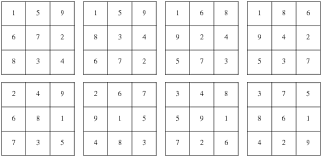### C Program For 3X3 Magic Square

What is magic square?

It is a 3x3 matrix in which we have to arrange numbers from 1-9 in such a way that the sum of all rows and columns is equal to 15.

Examples of magic square..Program for that in which user have to decide where to put 1.

#include<stdio.h>
#include<conio.h>

void main()
{
int a,i,j,c;
clrscr();

for(i=0;i<3;i++)
{
for(j=0;j<3;j++)
{
a[i][j]=0;
}
}
printf("\nEnter value of i and j:");
scanf("%d%d",&i,&j);
for(c=1;c<=9;c++)
{

if(i==-1)
{
i=2;
}
if(i==3)
{
i=0;
}
if(j==3)
{
j=0;
}

a[i][j]=c;
if(c%3==0)
{
i--;
}
else
{
i++;
j++;
}

}
for(i=0;i<3;i++)
{
for(j=0;j<3;j++)
{
printf("%d\t",a[i][j]);
}
printf("\n");
}
getch();

}

NOTE :- Value of i and j starts from 0.